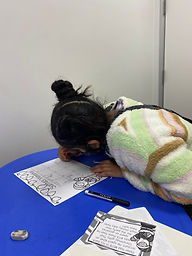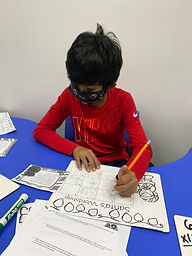Ms. Briana

Target 1​

Lesson Type:

Review

Number Operation

:

Computation

Solve multi-step word problems within 100 (addition, subtraction, multiplication, and division).

1:

Identify the correct arithmetic processes based on the information presented in word problems.

2:

Find the key words in word problems that indicate the correct arithmetic process to use.

3:

Find and use the needed information in a word problem in order to solve.

4:

Write an equation from a word problem with a variable included in the equation. Then solve for the variable.

3rd

Vocabulary:

Cubes Method

Activities:

Students were given a multi-step word problems and they raced to complete the complete the problem using CUBES method.

Whoever finished correctly and explained how they got the answer first picked the next word problem.Home Exploration

Guiding Questions:Absent Students:

Target 2

:

1:

Define perimeter.

2:

Understand that perimeter is the distance around a figure.

3:

Understand that perimeter can be found by adding the lengths of the sides of a figure.

3rd

Vocabulary:

Tailors Tape, Perimeter

Activities:

Studentes familiarized themselves with measurement tools.

Students were given a holiday tree print out and they used rulers and tailors tape to measure the length of all the sides.

Students then used those measurement to determine the perimeter of the tree.

Students then found the perieter of other items in the room.Home Exploration

Guiding Questions:Target 3

:

Vocabulary:

Activities:Home Exploration